## Algebra

Showing posts with label interest. Show all posts
Showing posts with label interest. Show all posts

### Ratio and Proportion ApplicationsWhen setting up proportions, be sure to be consistent.  Units for the numerators should be the same and units for the denominators should be the same as well. After obtaining an equation with two equal fractions, cross multiply.

Proportion Problem: If 2 out of 3 dentists prefer Crest toothpaste, how many prefer Crest out of 600 dentists surveyed?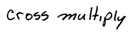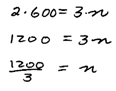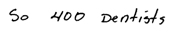Proportion Problem: In Visalia 3 out of every 7 voters said yes to proposition 40.  If 42,000 people voted, how many said no to proposition 40?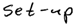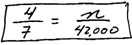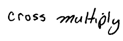Proportion Problem: A recipe calls for 5 tablespoons of sugar for every 8 cups of flour.  How many tablespoons of sugar are required for 32 cups of flour?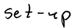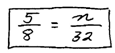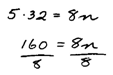Coin Problem: Sally has 12 coins consisting of quarters and dimes.  The value adds to \$2.25.  How many of each coin does she have?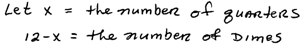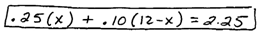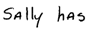Mixture Problem: A 50% alcohol solution is mixed with a 10% alcohol solution to create 8 ounces of a 32% alcohol solution.  How much of each is needed?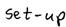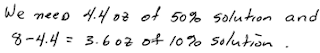Interest Problem: Mary invested her total savings of \$3,400 in two accounts.  Her mutual fund account earned 8% interest last year and her CD earned 5%.  If her total interest for the year was \$245, how much was in each account?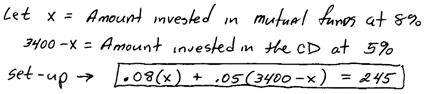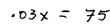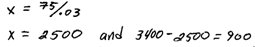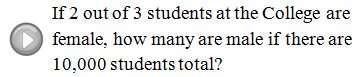### Applications of Linear Systems

Now that we have techniques for solving systems, we can set up our word problems with two variables.  If you use two variables, you will need to set up two equations.  With this in mind, look for two relationships and keywords when reading the questions.

Typical Word Problem: Last season two running backs on the Steelers football team rushed for a combined total of 1,550 yards.  One rushed 4 times as many yards as the other.  How many yards were rushed by each player?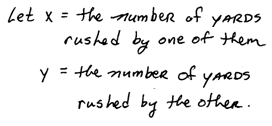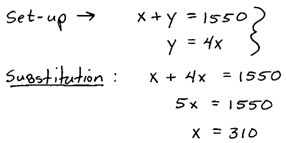Answer: One player rushed for 310 yards and the other rushed for 1,240 yards.

The set-up determines the method that we will choose to solve the system.  Since the y variable was isolated the easiest method to choose was the substitution method.  However, it does not matter which method we choose - the answer will be the same.

Typical Word Problem: A particular Algebra textbook has a total of 1,382 pages which is broken up into two parts.  The second part of the book has 64 more pages than the first part.  How many pages are in each part of the book?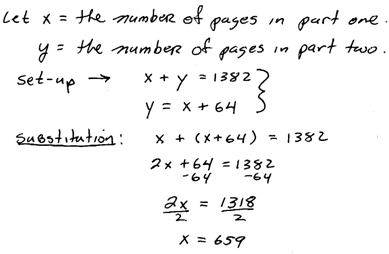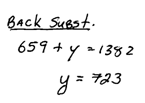Answer: There are 659 pages in the first part and 723 pages in the second.

Mixture Word Problem: Dennis mowed his next door neighbor's lawn for a handful of dimes and nickels, 80 coins in all.  Upon completing the job, he counted out the coins and it came to \$6.60.  How many of each coin did he earn?Mixture Word Problem: On Monday Joe bought 10 cups of coffee and 5 donuts for his office at the cost of \$16.50.  It turns out that the donuts were more popular than the coffee.  On Tuesday he bought 5 cups of coffee and 10 donuts for a total of \$14.25.  How much was each cup of coffee?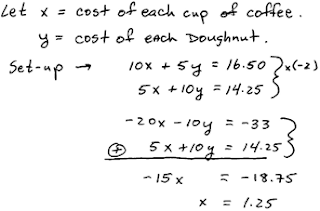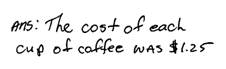Mixture Word Problem: A bartender wishes to mix an 8 ounce drink containing 20% alcohol.  He has two liquors, one with a 50% alcohol content and another with a 10% alcohol content.  How much of each liquor does he need to mix together?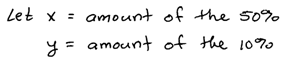The total alcohol content is 20% of 8 ounces. Now solve.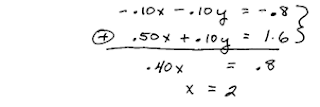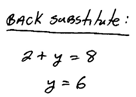Answer: He needs 2 ounces of the 50% alcohol liquor and 6 ounces of the 10% alcohol liquor.

Geometry Word Problem: Two angles are supplementary.  The larger angle is 48 degrees more than 10 times the smaller angle.  Find the measure of each angle. (Supplementary angle add to 180 degrees)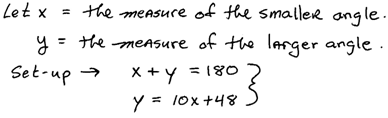Here we choose the substitution method.Answer: The two angles measure 12 degrees and 168 degrees.

Geometry Word Problem: Two angles are complementary.  The larger angle is 3 degrees less than twice the measure of the smaller angle.  Find the measure of each angle. (Complementary angles add to 90 degrees.)Here we choose the substitution method.Answer: The two angles measure 31 degrees and 59 degrees.

Perimeter Word Problem: The perimeter of a rectangular garden is 62 feet.  The length is 1 foot more than twice the width.  Find the dimensions of the garden.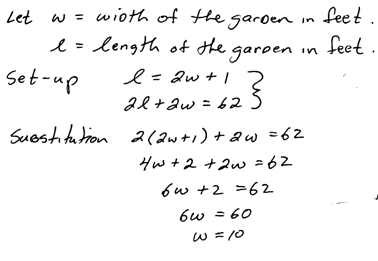Interest Word Problem: Sally's \$1,800 savings was in two accounts.  Her total interest for the year was \$93, where one account earned 6% annual interest and another earned 3%.  How much did she have in each account?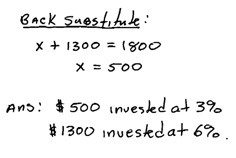When setting up these word problems look for totals.  The above is very typical, notice that one of the equations consists of the total amount invested, x + y = 1,800. The other equation represents the total amount of interest for the year, 0.03x + 0.06y = 93.  Two linear equations allow you to solve for the variables.

Also notice that it is wise to identify your variables every time.  This focuses your efforts and aids you in finding the solution.  It also tells you what our answers mean at the end.

Interest Word Problem: Millicent has \$10,000 invested in two accounts.  For the year she earned \$535 more in interest from her 7% Mutual Fund account than she did from her 4% CD.  How much does she have in each account?Always check to make sure your answer makes sense in terms of the word problem.  If you come up with an answer of say x = \$20,000 in the problem above you know this is unreasonable since the total amount is \$10,000.  At that point you should go back and check your set-up then check your algebra from there.

To solve distance problems, sometimes called uniform motion problems, it helps to organize the given data.  First identify the variables then try to fill in the chart with the appropriate values.  Sometimes your set up can come from columns in the chart and other times the set up will come from the rows.  Remember the formula D = r * t.

Uniform Motion Problem: An executive traveled 1,930 miles by car and by plane.  He drove to the airport at an average speed of 60 miles per hour and the plane averaged 350 miles per hour.  The total trip took 8 hours.  How long did it take to get to the airport?Uniform Motion Problem: A boat traveled 24 miles downstream in 2 hours.  The return trip took twice as long.  What is the speed of the boat in still water?Word problems take practice.  Be sure to do all of the assigned word problems and review them often.  Do not plan on skipping them on the exams - this is not a winning strategy.  Usually, once we set our word problems up correctly, the algebra is easier than other problems.